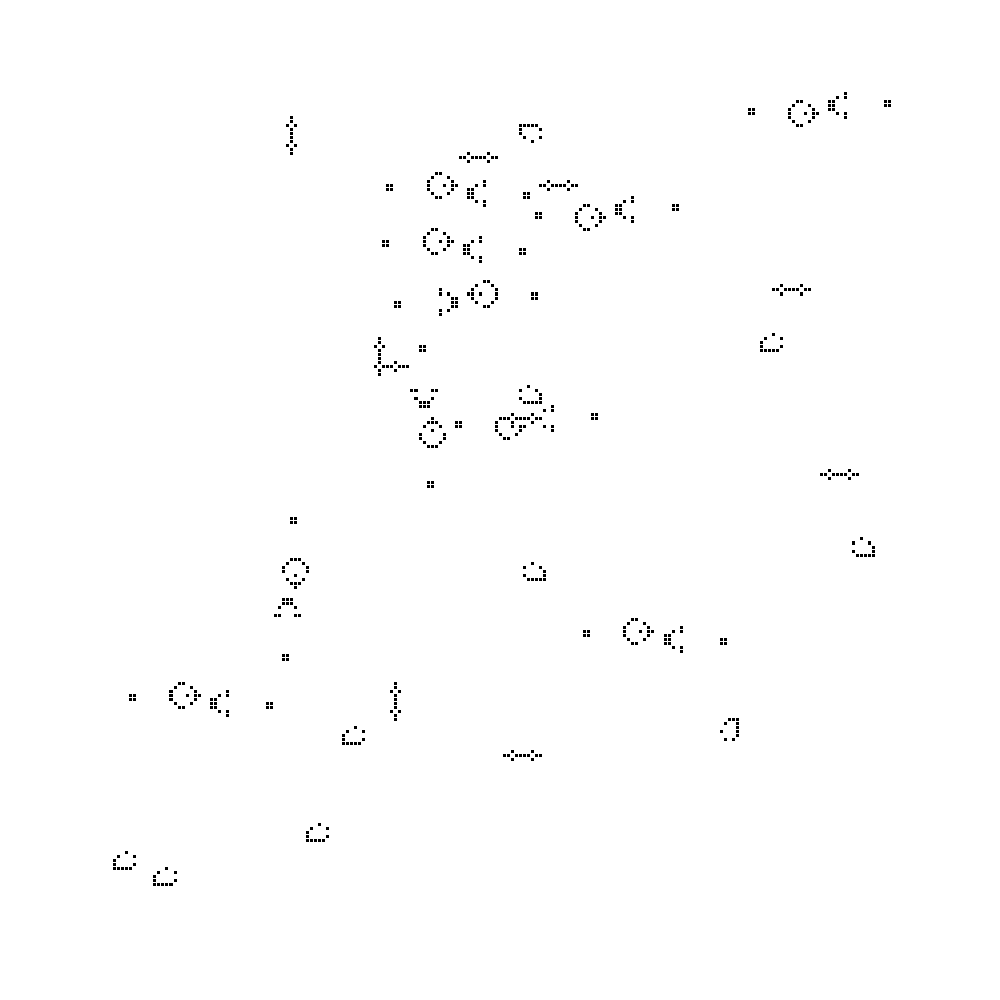# Conway’s Game of Life in R: Or On the Importance of Vectorizing Your R Code

October 28, 2018
By

Want to share your content on R-bloggers? click here if you have a blog, or here if you don't.

R is an interpreted programming language with vectorized data structures. This means a single R command can ask for very many arithmetic operations to be performed. This also means R computation can be fast. We will show an example of this using Conway’s Game of Life.

Conway’s Game of Life is one of the most interesting examples of cellular automata. It is traditionally simulated on a rectangular grid (like a chessboard) and each cell is considered either live or dead. The rules of evolution are simple: the next life grid is computed as follows:

• To compute the state of a cell on the next grid sum the number of live cells in the eight neighboring cells on the current grid.
• If this sum is 3 or if the current cell is live and the sum is 2 or 3, then the cell in the next grid will be live.

This rule can be implemented as scalar code in R:

```# d is a matrix of logical values
life_step_scalar <- function(d) {
nrow <- dim(d)[]
ncol <- dim(d)[]
dnext <- matrix(data = FALSE, nrow = nrow, ncol = ncol)
for(i in seq_len(nrow)) {
for(j in seq_len(ncol)) {
pop <- 0
if(i>1) {
if(j>1) {
pop <- pop + d[i-1, j-1]
}
pop <- pop + d[i-1, j]
if(j1) {
pop <- pop + d[i, j-1]
}
if(j1) {
pop <- pop + d[i+1, j-1]
}
pop <- pop + d[i+1, j]
if(j=2) && (pop<=3))
}
}
dnext
}
```

We could probably speed the above code up by a factor of 2 to 4 by eliminating the if-statements which requires writing 9 versions of the for-loops (depending if the index is in the right-boundary, interior, or left-boundary of its range). However, as we are about to see- this is not worth the effort.

A much faster implementation is the vector implementation found here.

```life_step <- function(d) {
# form the neighboring sums
nrow <- dim(d)[]
ncol <- dim(d)[]
d_eu <- rbind(d[-1, , drop = FALSE], 0)
d_ed <- rbind(0, d[-nrow, , drop = FALSE])
d_le <- cbind(d[ , -1, drop = FALSE], 0)
d_re <- cbind(0, d[ , -ncol, drop = FALSE])
d_lu <- cbind(d_eu[ , -1, drop = FALSE], 0)
d_ru <- cbind(0, d_eu[ , -ncol, drop = FALSE])
d_ld <- cbind(d_ed[ , -1, drop = FALSE], 0)
d_rd <- cbind(0, d_ed[ , -ncol, drop = FALSE])
pop <- d_eu + d_ed + d_le + d_re + d_lu + d_ru + d_ld + d_rd
d <- (pop==3) | (d & (pop>=2) & (pop<=3))
d
}
```

The way this code works is: it builds 8 copies of the life-table, one shifting each neighboring cell into the current cell-position. With these 8 new matrices the entire life forward evolution rule is computed vectorized over all cells using the expression “`(pop==3) | (d & (pop>=2) & (pop<=3))`“. Notice the vectorized code is actually shorter: we handle the edge-cases by zero-padding.

The performance difference is substantial:The vectorized code is about 10 times faster on average (details can be found here).

A simulation of this type produces figures such as the following:Of course if you are serious about Conway’s Game of Life you would use specialized software (even in-browser JavaScript), and specialized algorithms (such as HashLife).

One objection is: the vectorized code uses more memory. To that I give the following famous quote:

The biggest difference between time and space is that you can’t reuse time.

-Merrick Furst

And that is our (toy) example of vectorizing code. Techniques such as these are why very fast and powerful code can in fact be written in R.

R-bloggers.com offers daily e-mail updates about R news and tutorials about learning R and many other topics. Click here if you're looking to post or find an R/data-science job.
Want to share your content on R-bloggers? click here if you have a blog, or here if you don't.